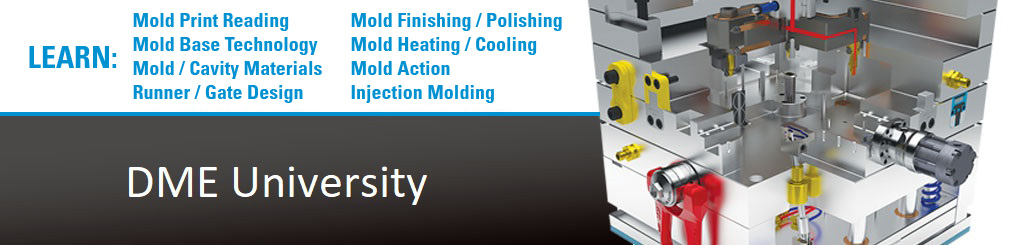# Convert an Inch Decimal to Metric

Pick a number from the Decimal column. Using your calculator, multiply it by the Inches-to-Metric conversion number 25.4 (because there are 25.4 Millimeters in an Inch).

The result is a Metric number (in Millimeters). It should match the respective number in the Metric column.

Previous

Next

Page 3 of 32